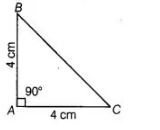# The area of a ΔABC is 8 cm2 in`
Question:

The area of a ΔABC is 8 cmin which AB = AC = 4 cm and ∠A = 90°.

Solution:

True

We have, ABC is a right angled triangle at A, where sides are given AB = AC = 4 cm

∴ Area of a triangle ABC = ½ (Base x Height) = ½ x AC x AB=½ x 4 x 4=8 cm2